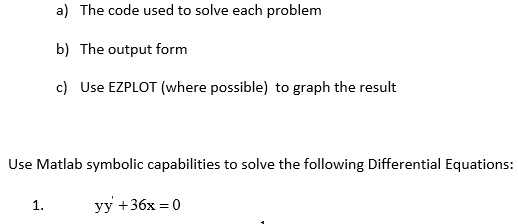# How Graph differential equations with Matlab

509 ビュー (過去 30 日間)
jake thompson 2018 年 1 月 17 日
Commented: Rena Berman 2019 年 12 月 12 日
Looking to get some help on how to use matlab to solve the following equation problem 1, all help is appreciated! Thank You! I haven't used matlab in 2 years very rusty, image is reference to original problem statement. List of equations%%PROBLEM 1
syms y(x)
ode = y*diff(y,x)+36*x == 0;
ySol(x) = dsolve(ode)
ezplot(y(x))

#### 1 件のコメント

Rena Berman 2019 年 12 月 12 日

サインイン to comment.

### 採用された回答

Star Strider 2018 年 1 月 17 日
The integrated equations produce results that are pure imaginary. You have to plot the real and imaginary parts of each solution separately with ezplot. You also have to define the initial condition, y(0).
Try this:
syms y(x)
ode = y*diff(y,x)+36*x == 0;
ySol = dsolve(ode, y(0) == 0)
figure
subplot(2,1,1)
ezplot(real(ySol(1)))
subplot(2,1,2)
ezplot(imag(ySol(1)))
figure
subplot(2,1,1)
ezplot(real(ySol(2)))
subplot(2,1,2)
ezplot(imag(ySol(2)))

#### 2 件のコメント

jake thompson 2018 年 1 月 18 日
Thank you, it looks like it is working on all my first order equations. Would you do something different if you had a second order differential equation?
Star Strider 2018 年 1 月 18 日
Yes, if the second-order differential equation required it.

サインイン to comment.